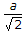# Mechanical Engineering - Theory of machines

### Exercise :: Theory of machines - Section 2

1.

The maximum or minimum value of the swaying couple is

 A. ± c.m.ω2.r B. ± a(1 - c)m.ω2.r C. ±(1 - c)m.ω2.r D. ± 2a(1 - c)m.ω2.r

Explanation:

No answer description available for this question. Let us discuss.

2.

For an involute gear, the ratio of base circle radius and pitch circle radius is equal to

 A. sin φ B. cos φ C. sec φ D. cosec φ

Explanation:

No answer description available for this question. Let us discuss.

3.

A chain consisting of four links and four joints is called a kinematic chain.

 A. Yes B. No

Explanation:

No answer description available for this question. Let us discuss.

4.

Which of the following is an open pair?

 A. Ball and socket joint B. Journal bearing C. Lead screw and nut D. Cam and follower

Explanation:

No answer description available for this question. Let us discuss.

5.

The frictional torque transmitted in a flat pivot bearing with assumption of uniform pressure is __________ as compared to uniform wear.

 A. less B. more C. same